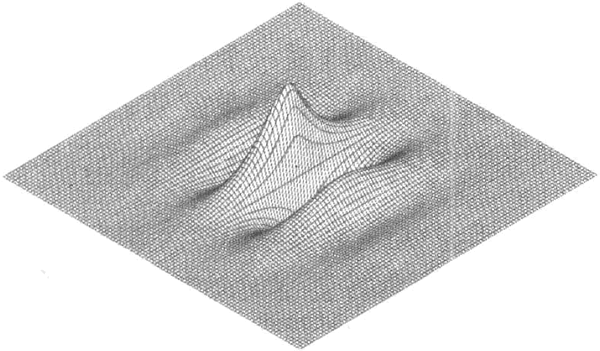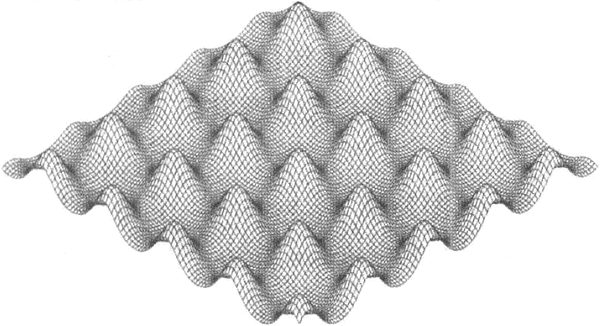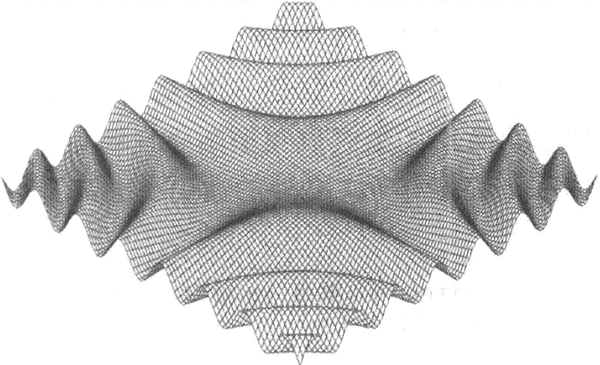## Go语言表达式求值器

• 内容
• 评论
• 相关

// Expr：算术表达式
type Expr interface{}

sqrt(A / pi)
pow(x, 3) + pow(y, 3)
(F - 32) * 5 / 9

```// Var 表示一个变量，比如 x
type Var string
// literal 是一个数字常量，比如 3.141
type literal float64
// unary 表示一元操作符表达式，比如-x
type unary struct {
op rune // '+', '-' 中的一个
x Expr
}
// binary 表示二元操作符表达式，比如 x+y
type binary struct {
op rune // '+', '-', '*', '/' 中的一个
x, y Expr
}
// call 表示函数调用表达式，比如 sin(x)
type call struct {
fn string // one of "pow", "sin", "sqrt" 中的一个
args []Expr
}```

type Env map[Var]float64

type Expr interface {
// Eval 返回表达式在 env 上下文下的值
Eval(env Env) float64
}

func (v Var) Eval(env Env) float64 {
return env[v]
}
func (l literal) Eval(_ Env) float64 {
return float64(l)
}

unary 和 binary 的 Eval 方法首先对它们的操作数递归求值，然后应用 op 操作。我们不把除以 0 或者无穷大当做错误（尽管它们生成的结果显然不是有穷数）。最后，call 方法先对 pow、sin 或者 sqrt 函数的参数求值，再调用 math 包中的对应函数。

```func (u unary) Eval(env Env) float64 {
switch u.op {
case '+':
return +u.x.Eval(env)
case '-':
return -u.x.Eval(env)
}
panic(fmt.Sprintf("unsupported unary operator: %q", u.op))
}
func (b binary) Eval(env Env) float64 {
switch b.op {
case '+':
return b.x.Eval(env) + b.y.Eval(env)
case '-':
return b.x.Eval(env) - b.y.Eval(env)
case '*1:
return b.x.Eval(env) * b.y.Eval(env)
case '/':
return b.x.Eval(env) / b.y.Eval(env)
}
panic(fmt.Sprintf("unsupported binary operator: %q", b.op))
}
func (c call) Eval(env Env) float64 {
switch c.fn {
case "pow":
return math.Pow(c.args.Eval(env), c.args.Eval(env)
case "sin":
return math.Sin(c.args.Eval(erw))
case "sqrt":
return math.Sqrt(c.args.Eval(env))
}
panic(fmt.Sprintf("unsupported function call: %s", c.fn))
}```

```func TestEval(t *testing.T) {
tests := []struct {
expr string
env Env
want string
}{
{"sqrt(A / pi)", Env{"A": 87616, "pi": math.Pi}, "167"},
{"pow(x, 3) + pow(y, 3)", Env{"x": 12, "y": 1}, "1729"},
{"pow(x, 3) + pow(y, 3)", Env{"x": 9, "y": 10}, "1729"},
{"5/9 * (F - 32)", Env{"F": -40}, "-40"},
{"5/9 * (F - 32)", Env{"F": 32}, "0"},
{"5/9 * (F - 32)", Env{"F": 212}, "100"},
}
var prevExpr string
for _, test := range tests {
// 仅在表达式变更时才输出
if test.expr != prevExpr {
fmt.Printf("\n%s\n", test.expr)
prevExpr = test.expr
}
expr, err := Parse(test.expr)
if err != nil {
t.Error(err) // 解析出错
continue
}
got := fmt.Sprintf("%.6g", expr.Eval(test.env))
fmt.Printf("\t%v => %s\n", test.env, got)
if got != test.want {
t.Errorf("%s.Eval() in %v = %q, want %q\n", test.expr, test.env, got, test.want)
}
}
}```

go test 命令可用于运行包的测试：

\$ go test -v gopl.io/ch7/eval

sqrt(A / pi)
map[A:87616 pi:3.141592653589793] => 167

pow(x, 3) + pow(y, 3)
map[x:12 y:1] => 1729
map[x:9 y:10] => 1729
5 / 9 * (F - 32)
map[F:-40] => -40
map[F:32] => 0
map[F:212] => 100

type Expr interface {
Eval(env Env) float64
// Check 方法报告表达式中的错误，并把表达式中的变量加入 Vars 中
Check(vars map[Var]bool) error
}

```func (v Var) Check(vars map[Var]bool) error {
vars[v] = true
return nil
}
func (literal) Check(vars map[Var]bool) error {
return nil
}
func (u unary) Check(vars map[Var]bool) error {
if !strings.ContainsRune("+-", u.op) {
return fmt.Errorf("unexpected unary op %q", u.op)
}
return u.x.Check(vars)
}
func (b binary) Check(vars map[Var]bool) error {
if !strings.ContainsRune("+-*/", b.op) {
return fmt.Errorf("unexpected binary op %q", b.op)
}
if err := b.x.Check(vars); err != nil {
return err
}
return b.y.Check(vars)
}
func (c call) Check(vars map[Var]bool) error {
arity, ok := numParams[c.fn]
if !ok {
return fmt.Errorf("unknown function %q", c.fn)
}
if len(c.args) != arity {
return fmt.Errorf("call to %s has %d args, want %d", c.fn, len(c.args), arity)
}
for _, arg := range c.args {
if err := arg.Check(vars); err != nil {
return err
}
}
return nil
}
var numParams = map[string]int{"pow",: 2, "sin": 1, "sqrt": 1}```

x % 2  unexpected '%'
math.Pi unexpected '.'
!true  unexpected '!'
"hello" unexpected '"'
log(10) unknown function "log"
sqrt(1, 2) call to sqrt has 2 args, want 1

Check 的输入参数是一个 Ver 集合，它收集在表达中发现的变量名。要让表达式能成功求值，上下文必须包含所有的这些变量。从逻辑上来讲，这个集合应当是 Check 的输出结果而不是输入参数，但因为这个方法是递归调用的，在这种情况下使用参数更为方便。调用方在最初调用时需要提供一个空的集合。

```import "gopl.io/ch7/eval"
func parseAndCheck(s string) (eval.Expr, error) {
if s == "" {
return nil, fmt.Errorf("empty expression")
}
expr, err := eval.Parse(s)
if err != nil {
return nil, err
}
vars := make(map[eval.Var]bool)
if err := expr.Check(vars); err != nil {
return nil, err
}
for v := range vars {
if v != "x" && v != "y" && v != "r" {
return nil, fmt.Errorf("undefined variable: %s", v)
}
}
return expr, nil
}```

```func plot(w http.ResponseWriter, r *http.Request) {
r.ParseForm()
expr, err := parseAndCheck(r.Form.Get("expr"))
if err != nil {
return
}
surface(w, func(x, y float64) float64 {
r := math.Hypot(x, y)   // 与(0,0)之间的距离
return expr.Eval(eval.Env{"x": x, "y": y, "r" : r})
})
}```

plot 函数解析并检查 HTTP 请求中的表达式，并用它来创建一个有两个变量的匿名函数。这个匿名函数与原始曲面图绘制程序中的f有同样的签名，且能对用户提供的表达式进行求值。上下文定义了 x、y 和半径 r。（a）(b)(c)

0条评论